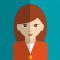## How to display a variable as tooltip in ggplotly using R language?Vani Nalliappan

Updated on 26-Oct-2022 11:58:52

R is a programming language for statistical computing and graphics. ggplotly() is a function used to convert static plots to web-based plots. ggplotly() returns a Plotly object. In this tutorial, we will see how to display a variable as tooltip in ggplotly using R language. Here, we will use ... Read More

## How to show multiple ggplot2 plots with Plotly using R?Vani Nalliappan

Updated on 26-Oct-2022 11:56:55

R is a programming language for statistical computing and graphics. ggplotly() is a function used to convert static plots to web-based plots. ggplotly() returns a Plotly object. In this tutorial, we will see how to show multiple ggplot2 plots with Plotly using R. Here, we will use the aes() ... Read More

## How to remove option bar from ggplotly using R?Vani Nalliappan

Updated on 26-Oct-2022 11:55:03

R is a programming language for statistical computing and graphics. ggplotly() is a function that is used to convert a static plot to an interactive web-based version. ggplotly() returns a Plotly object. In this tutorial, we will see how to remove the option bar from ggplotly using R. Here, ... Read More

## How to format mouse over labels using ggplotly in R?Vani Nalliappan

Updated on 26-Oct-2022 11:52:04

R is a programming language for statistical computing and graphics. ggplotly() is a function that is used to convert a static plot to an interactive web-based version. ggplotly() returns a Plotly object. In this tutorial, we will see how to format mouse over labels using ggplotly in R. Here, ... Read More

## How to plot on secondary Y-Axis with Python Plotly?Vani Nalliappan

Updated on 26-Oct-2022 11:44:38

Plotly is an open-source, interactive, and browser-based charting library for Python. Python users can use Plotly to generate different types of charts including scientific charts, 3D graphs, statistical charts, financial charts, etc. In this tutorial, we will show how you can use Plotly to plot data on the secondary Y-Axis. ... Read More

## How to add multiple text labels from DataFrame columns in Python Plotly?Vani Nalliappan

Updated on 26-Oct-2022 11:42:49

Plotly is an open-source plotting library in Python that can generate several different types of charts. Python users can use Plotly to create interactive web-based visualizations. In this tutorial, we will see how you can use Plotly to add multiple text labels in a chart from DataFrame columns. Here, ... Read More

## How to add multiple graphs to a Plotly Dash app on a single browser page in Python Plotly?Vani Nalliappan

Updated on 21-Oct-2022 09:37:33

Plotly is an open-source plotting library in Python that can generate several different types of charts. Python users can use Plotly to create interactive web-based visualizations that can be displayed in Jupyter notebooks, saved to standalone HTML files, or served as a part of web applications using Dash. Plotly can ... Read More

## Python Plotly – How to hide legend entries in a Plotly figure?Vani Nalliappan

Updated on 21-Oct-2022 09:33:23

Plotly is an open-source plotting library in Python that can generate several different types of charts. Python users can use Plotly to create interactive web-based visualizations including scientific charts, 3D graphs, statistical charts, financial charts, etc. Plotly can also be used in static document publishing and desktop editors such as ... Read More

## How to shade a chart above a specific Y value in Python Plotly?Vani Nalliappan

Updated on 21-Oct-2022 09:31:44

Plotly is an open-source plotting library in Python that can generate several different types of charts. Python users can use Plotly to generate different types of charts including scientific charts, 3D graphs, statistical charts, financial charts, etc. In this tutorial, we will show how you can use Plotly to shade ... Read More

## Python Plotly – How to change variable/label names for the legend in a line chart?Vani Nalliappan

Updated on 21-Oct-2022 09:27:32

Plotly is an open-source, interactive, and browser-based charting library for Python. Python users can use Plotly to generate different types of charts including scientific charts, 3D graphs, statistical charts, financial charts, etc. In this tutorial, we will show how you can use Plotly to change the variable and label names ... Read More

1 2 3 4 5 ... 14 Next